Successfully reported this slideshow.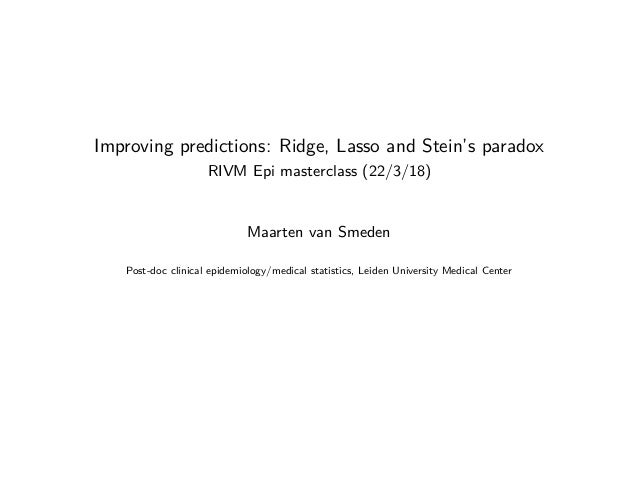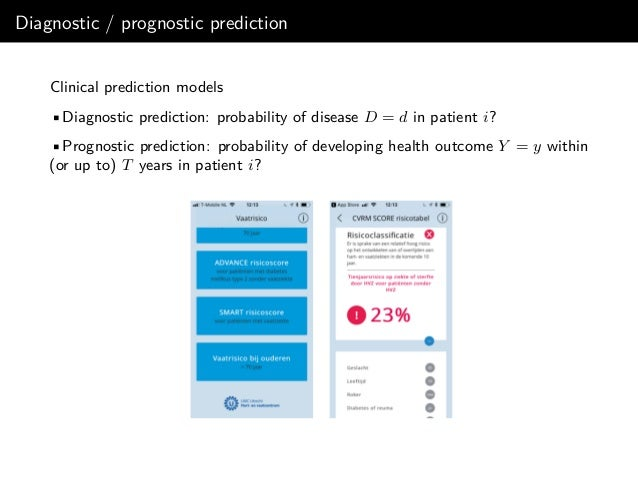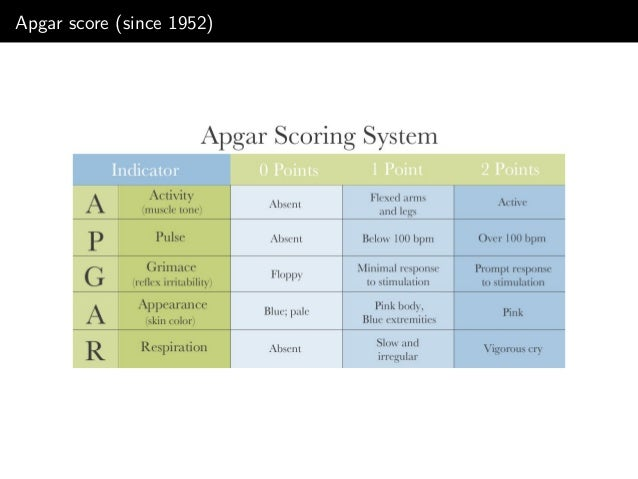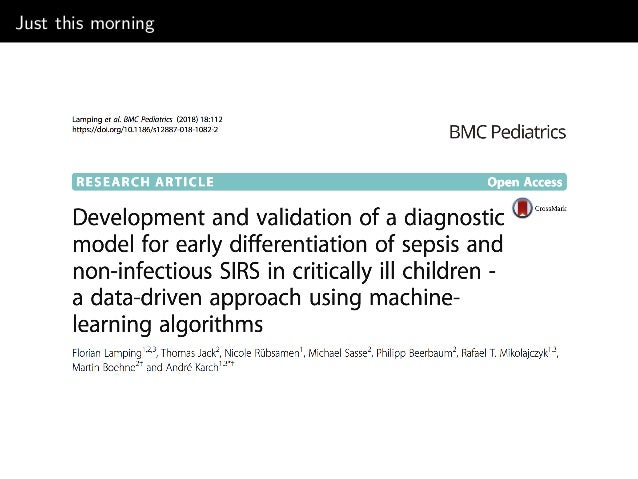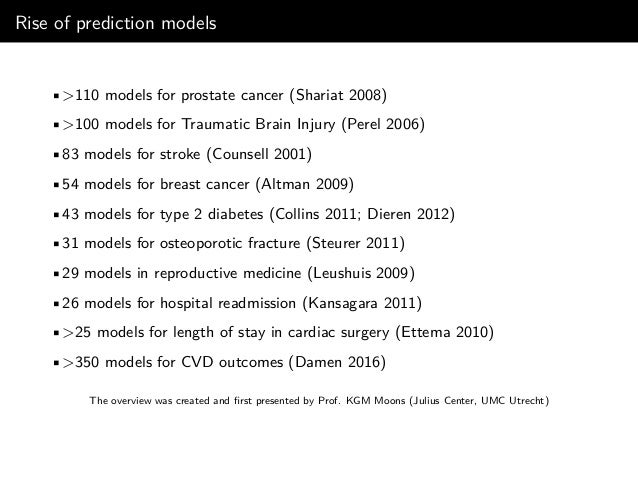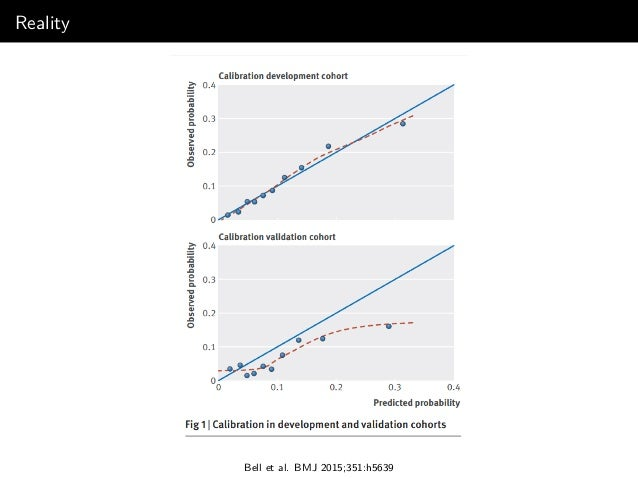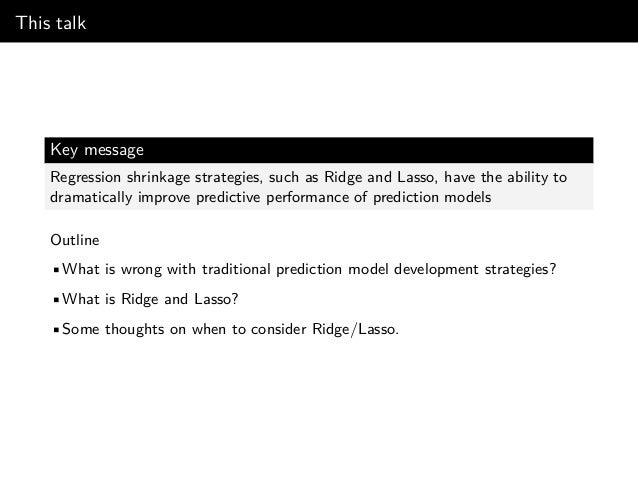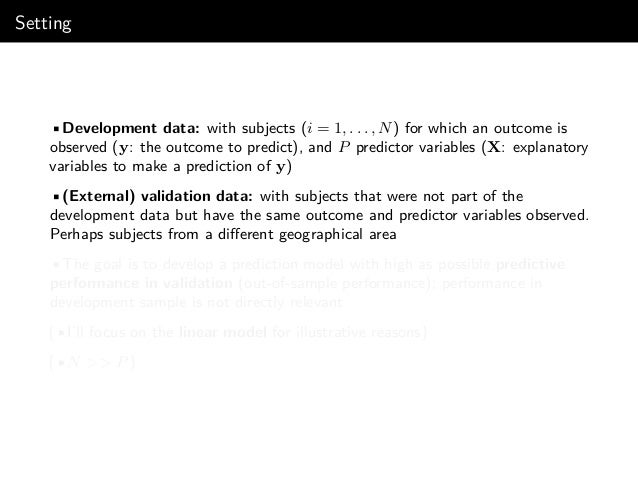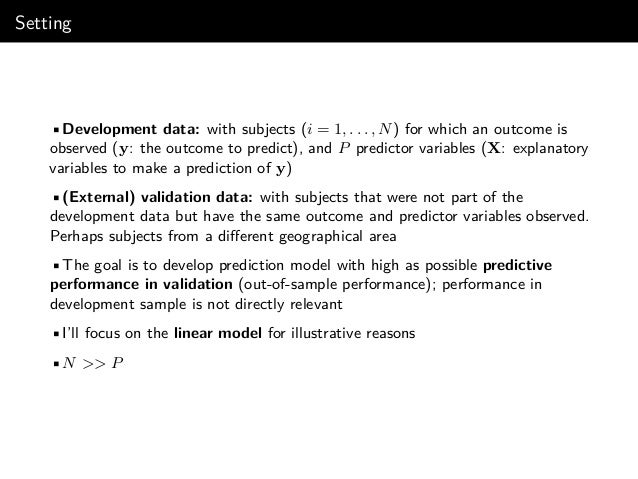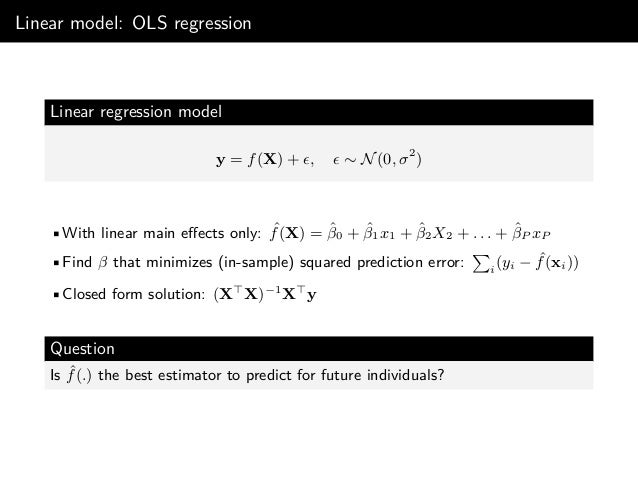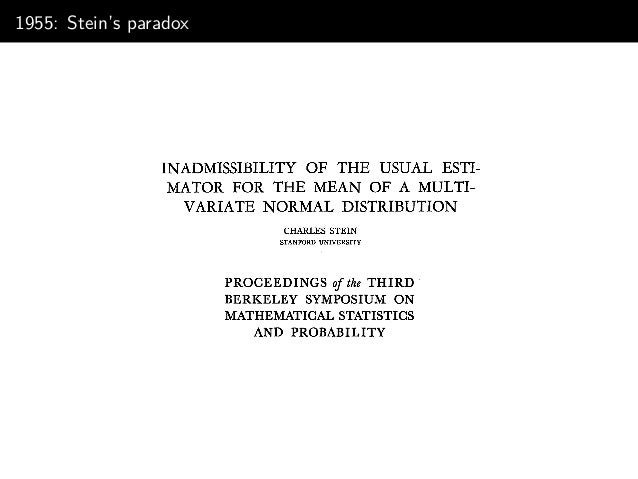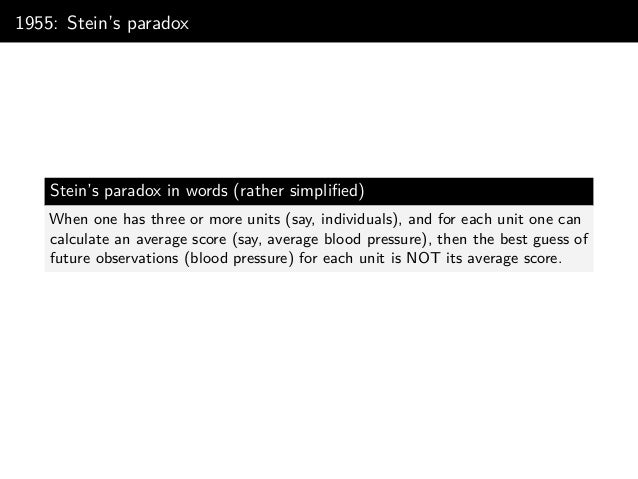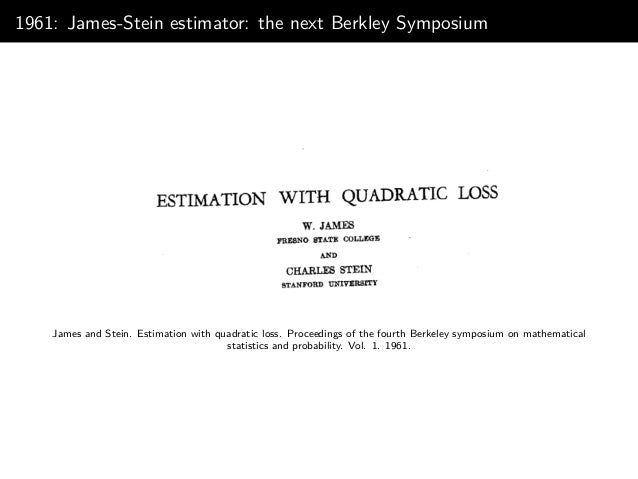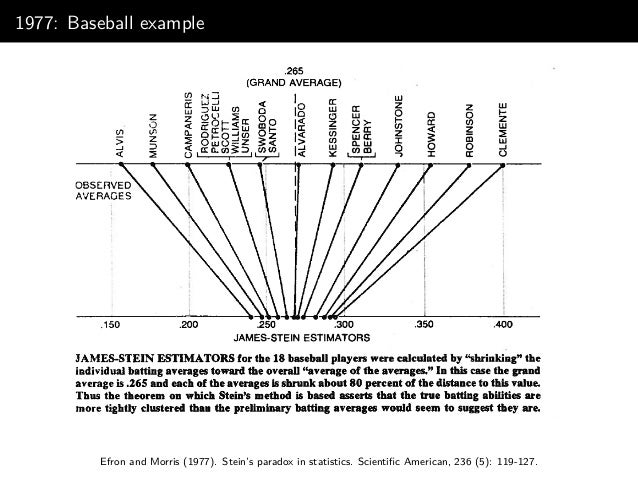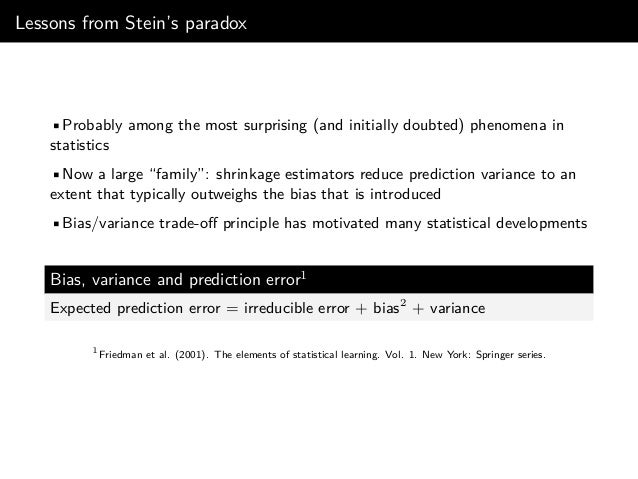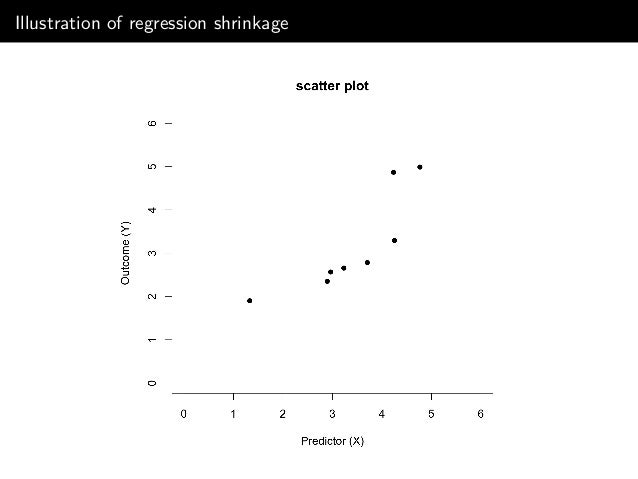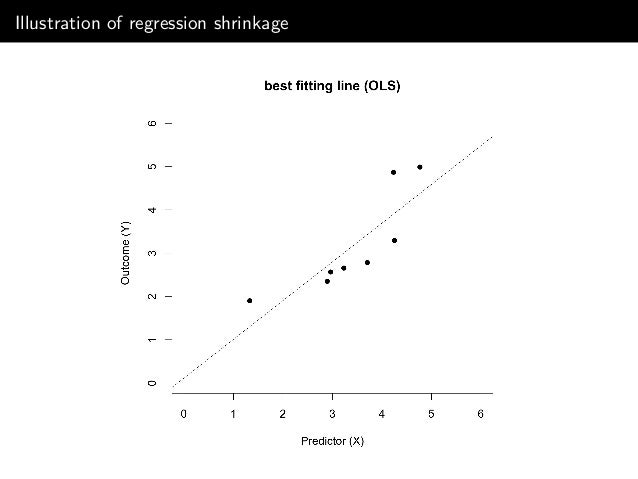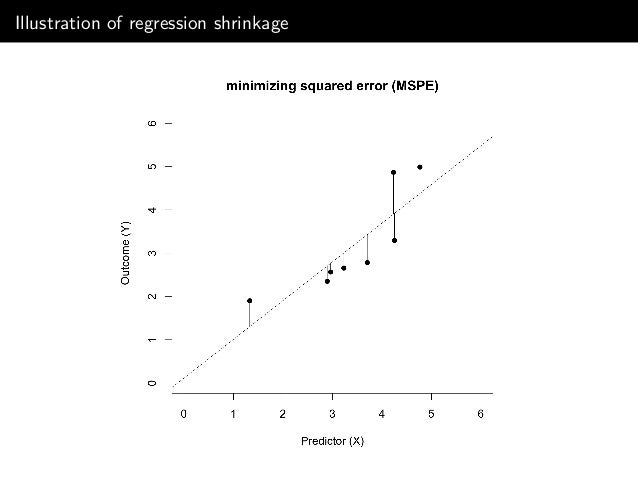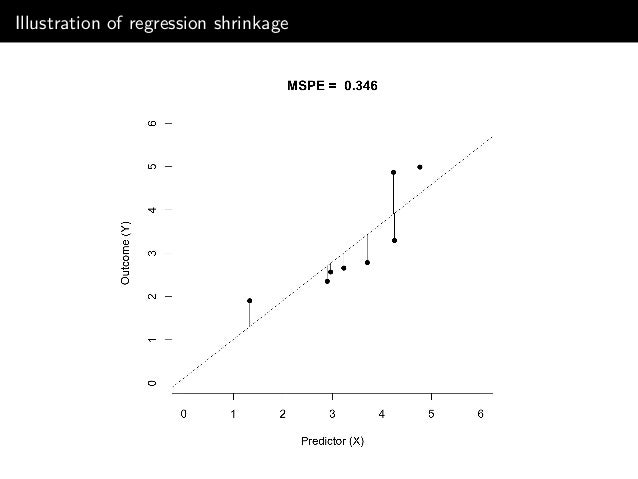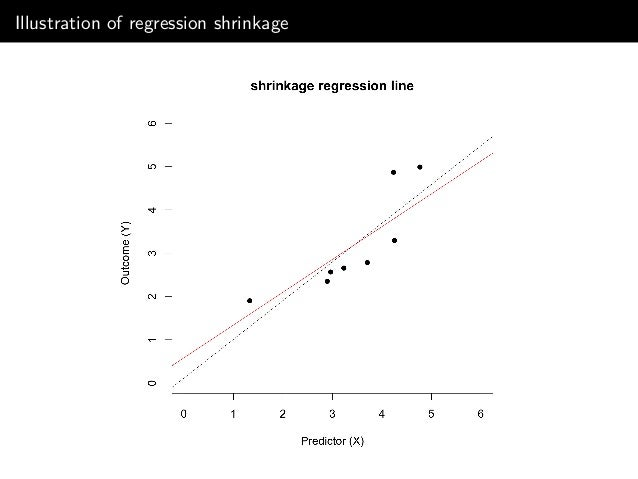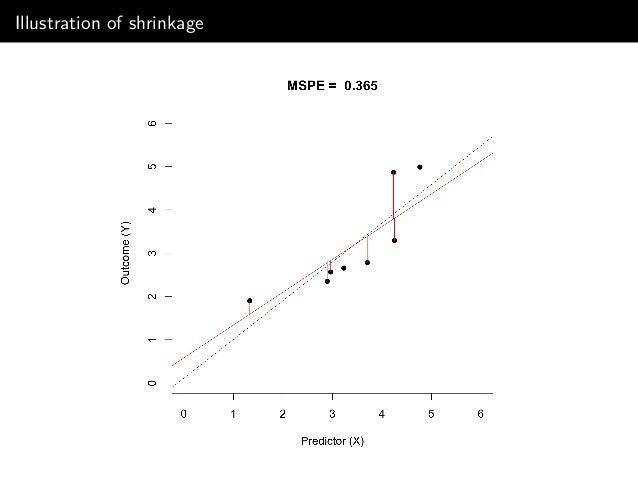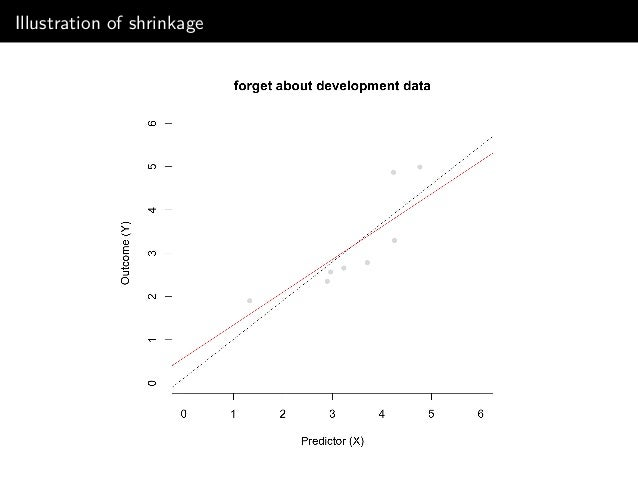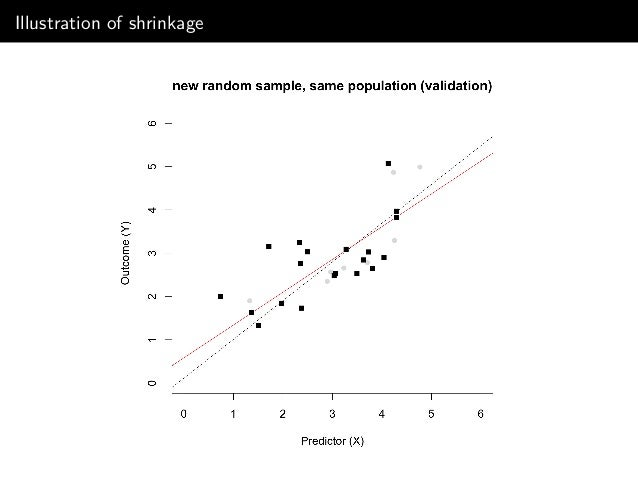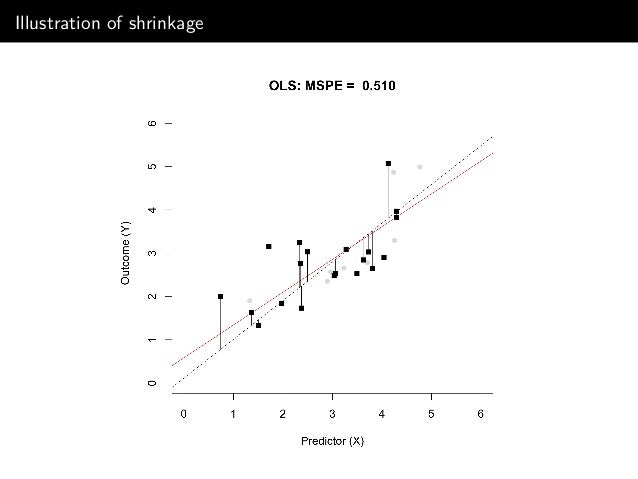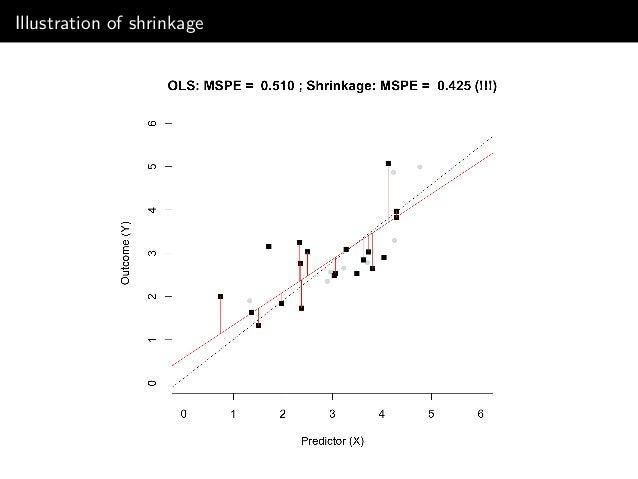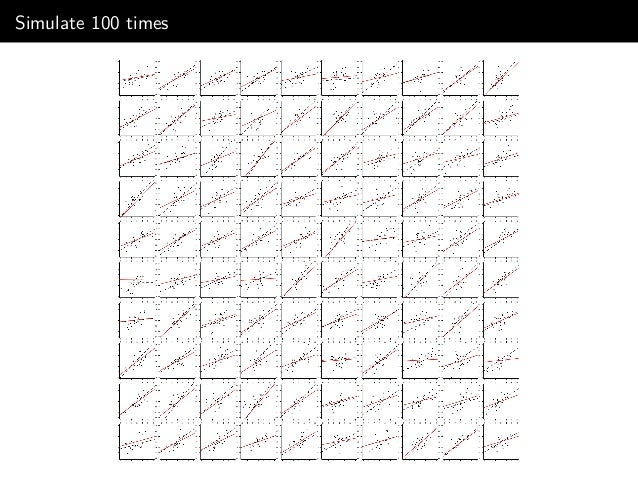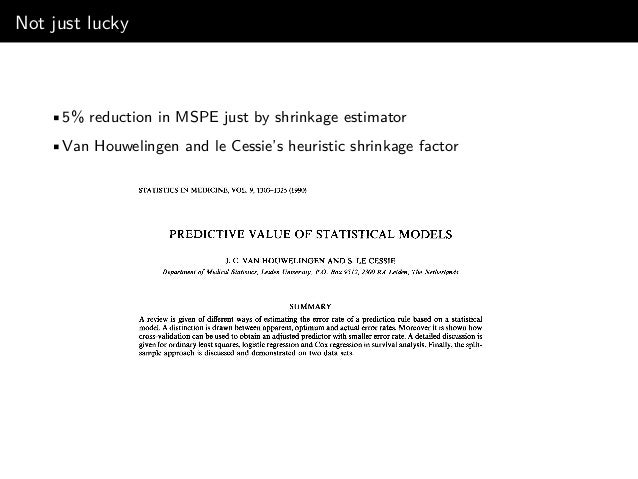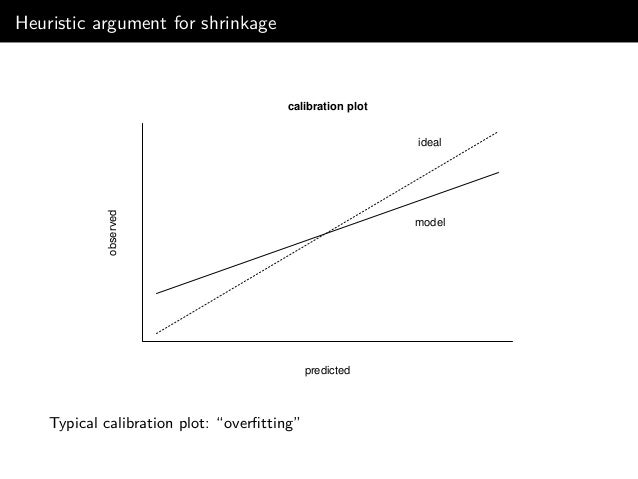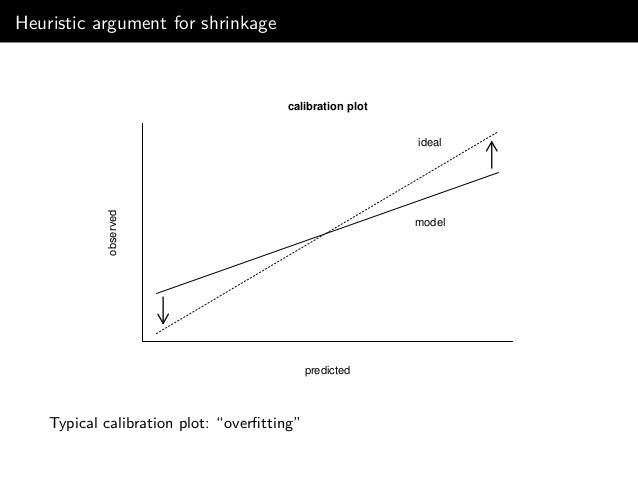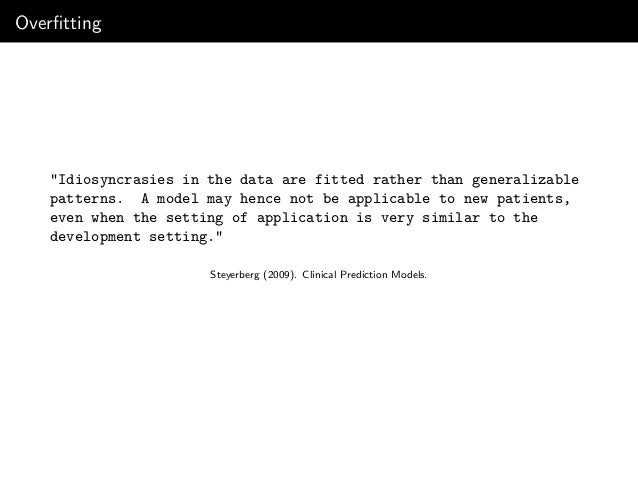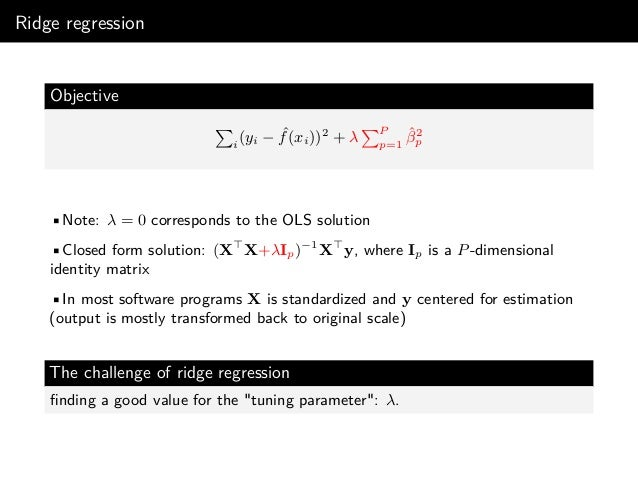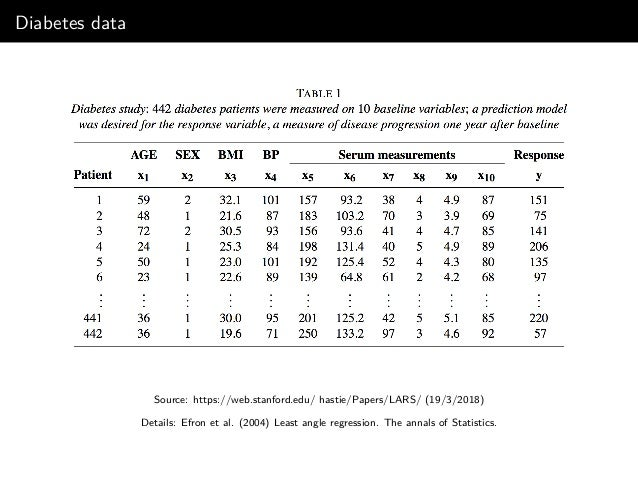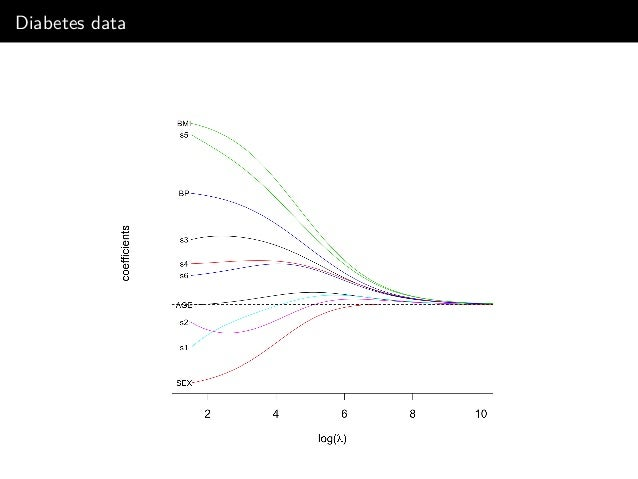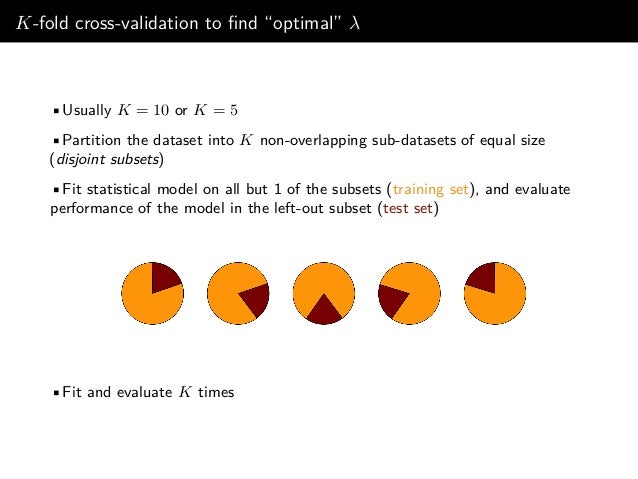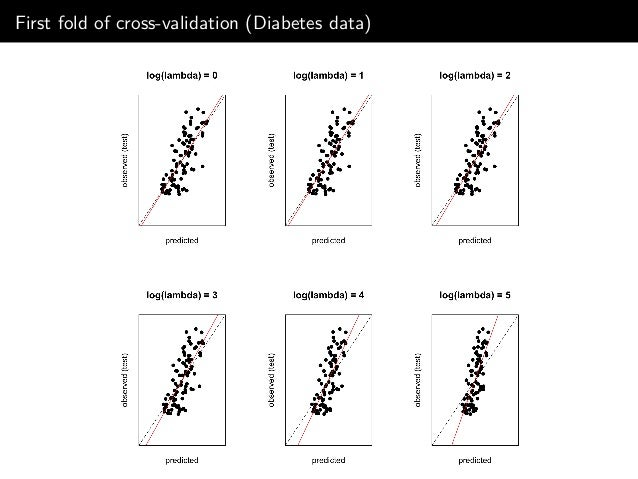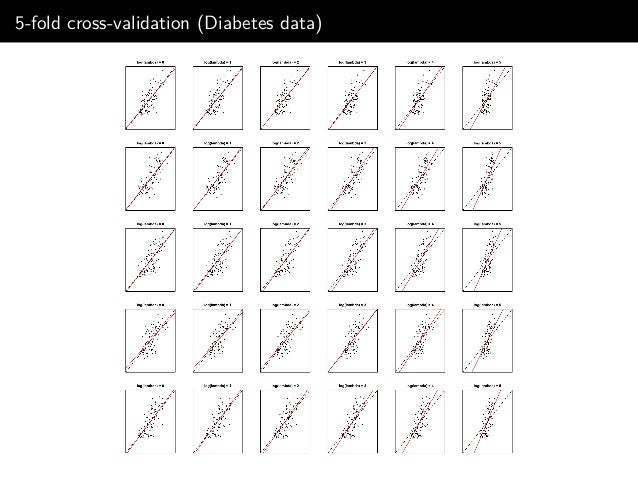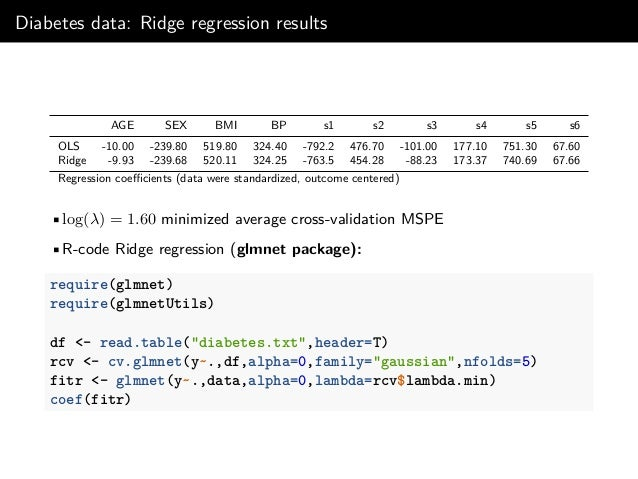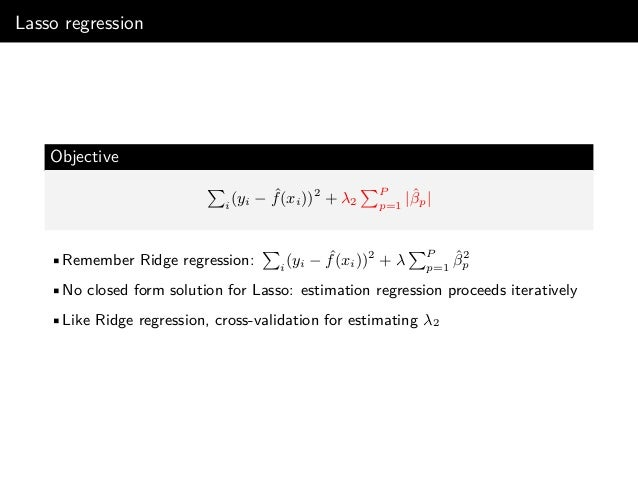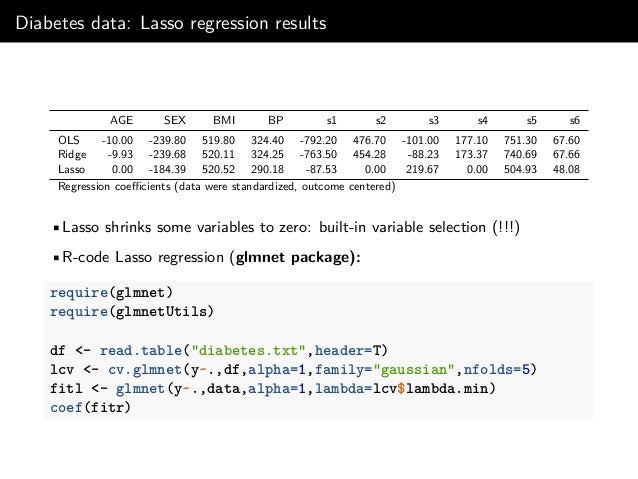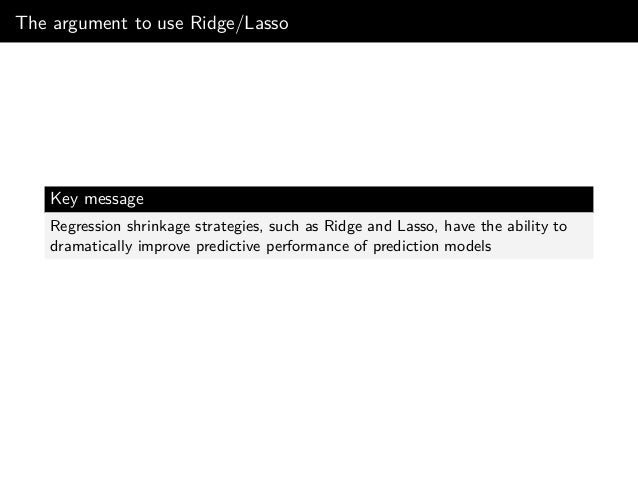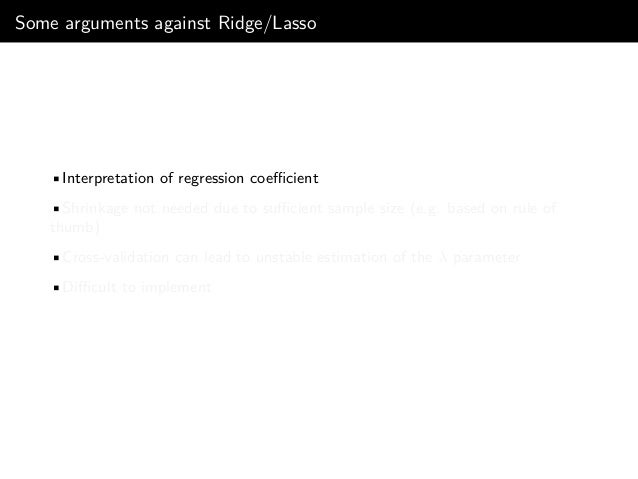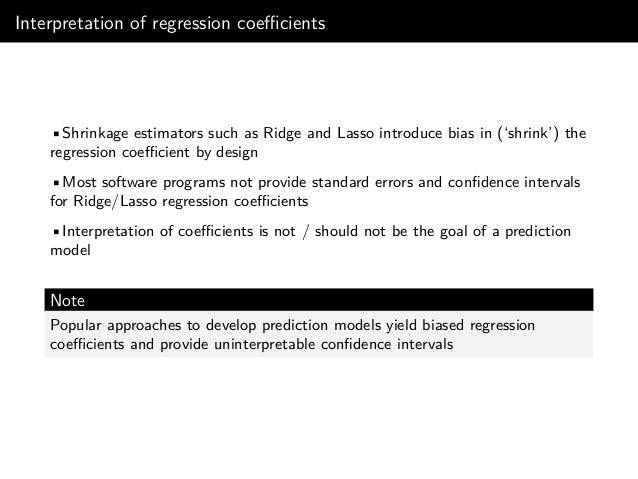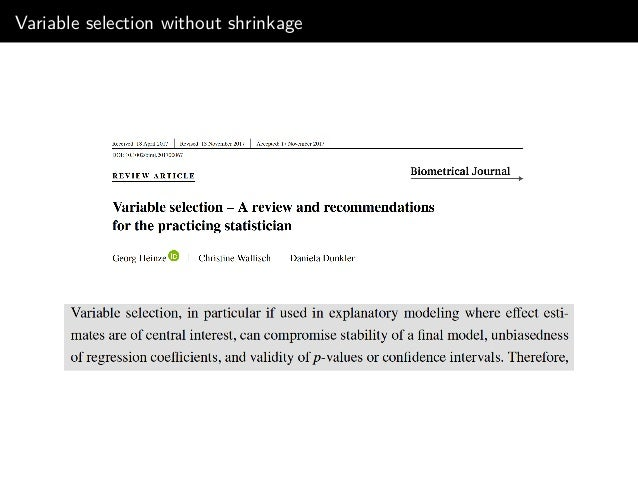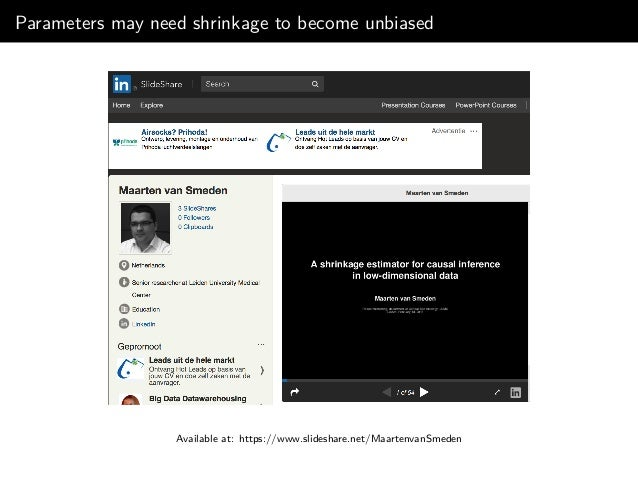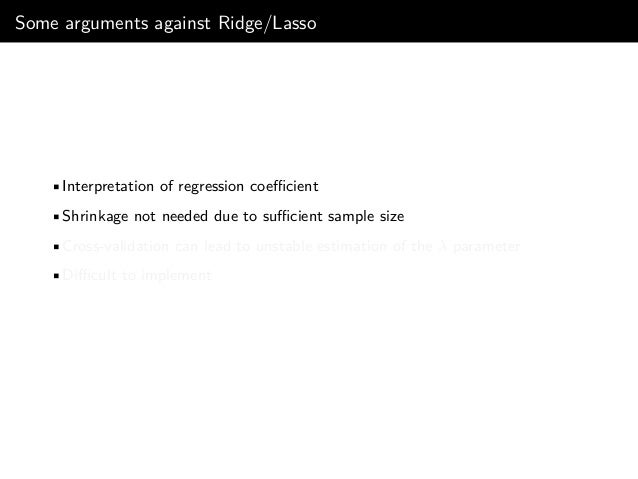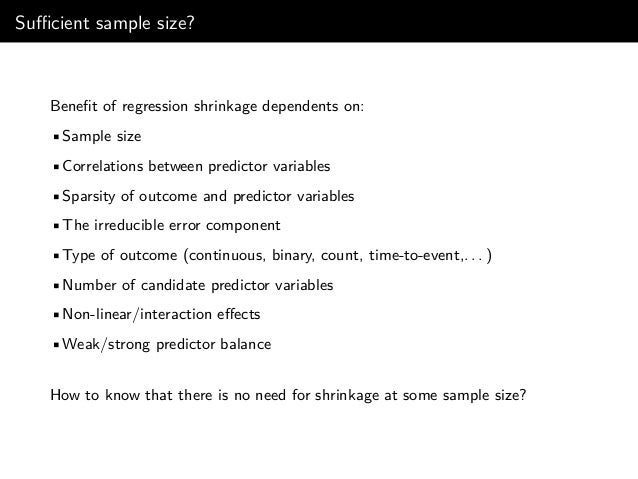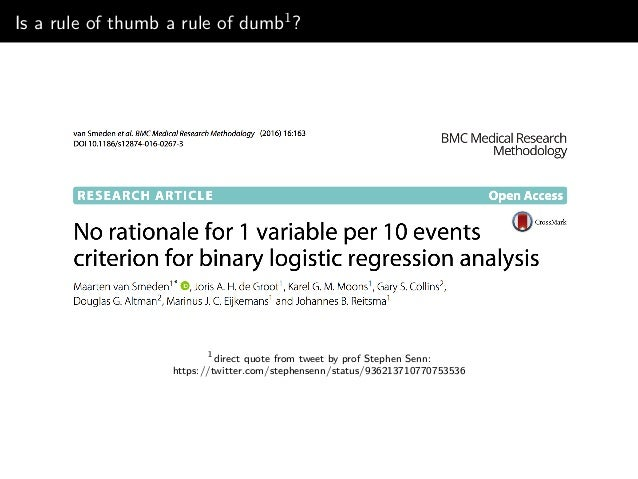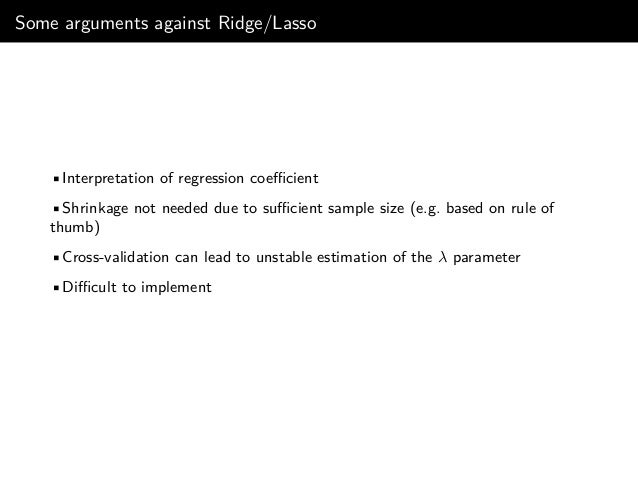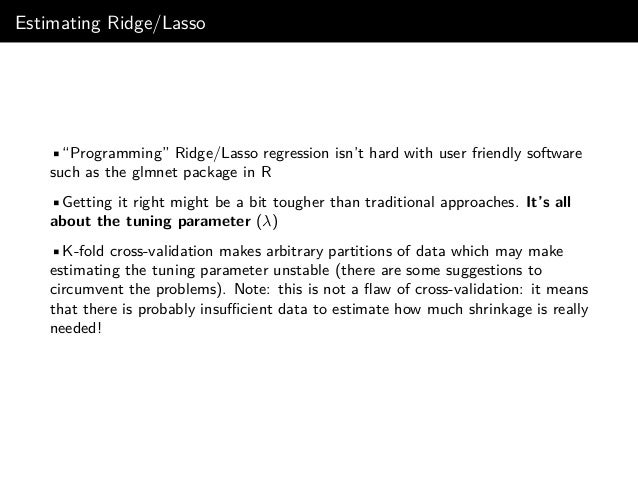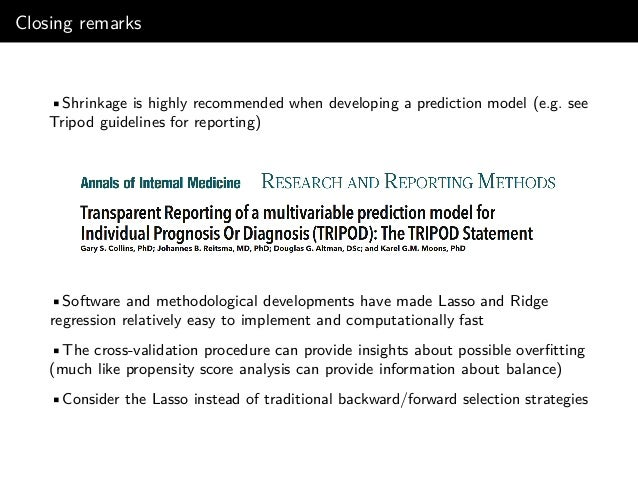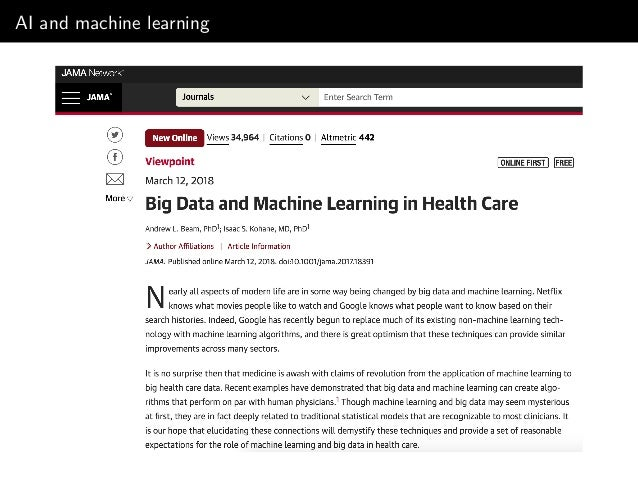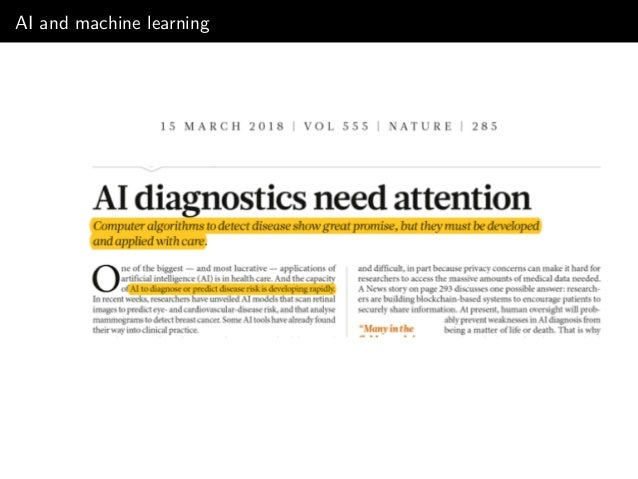Upcoming SlideShare
×

# Improving predictions: Lasso, Ridge and Stein's paradox

Slides of masterclass "Improving predictions: Lasso, Ridge and Stein's paradox" at the (Dutch) National Institute for Public Health and the Environment (RIVM)

• Full Name
Comment goes here.

Are you sure you want to Yes No• Be the first to comment

• Be the first to like this

### Improving predictions: Lasso, Ridge and Stein's paradox

1. 1. Improving predictions: Ridge, Lasso and Stein’s paradox RIVM Epi masterclass (22/3/18) Maarten van Smeden Post-doc clinical epidemiology/medical statistics, Leiden University Medical Center
2. 2. This slide deck available: https://www.slideshare.net/MaartenvanSmeden
3. 3. Diagnostic / prognostic prediction Clinical prediction models •Diagnostic prediction: probability of disease D = d in patient i? •Prognostic prediction: probability of developing health outcome Y = y within (or up to) T years in patient i?
4. 4. Apgar score (since 1952)
5. 5. Just this morning
6. 6. Rise of prediction models •>110 models for prostate cancer (Shariat 2008) •>100 models for Traumatic Brain Injury (Perel 2006) •83 models for stroke (Counsell 2001) •54 models for breast cancer (Altman 2009) •43 models for type 2 diabetes (Collins 2011; Dieren 2012) •31 models for osteoporotic fracture (Steurer 2011) •29 models in reproductive medicine (Leushuis 2009) •26 models for hospital readmission (Kansagara 2011) •>25 models for length of stay in cardiac surgery (Ettema 2010) •>350 models for CVD outcomes (Damen 2016) The overview was created and ﬁrst presented by Prof. KGM Moons (Julius Center, UMC Utrecht)
7. 7. Reality Bell et al. BMJ 2015;351:h5639
8. 8. This talk Key message Regression shrinkage strategies, such as Ridge and Lasso, have the ability to dramatically improve predictive performance of prediction models Outline •What is wrong with traditional prediction model development strategies? •What is Ridge and Lasso? •Some thoughts on when to consider Ridge/Lasso.
9. 9. Setting •Development data: with subjects (i = 1, . . . , N) for which an outcome is observed (y: the outcome to predict), and P predictor variables (X: explanatory variables to make a prediction of y) •(External) validation data: with subjects that were not part of the development data but have the same outcome and predictor variables observed. Perhaps subjects from a diﬀerent geographical area •The goal is to develop a prediction model with high as possible predictive performance in validation (out-of-sample performance); performance in development sample is not directly relevant {•I’ll focus on the linear model for illustrative reasons} {•N >> P}
10. 10. Setting •Development data: with subjects (i = 1, . . . , N) for which an outcome is observed (y: the outcome to predict), and P predictor variables (X: explanatory variables to make a prediction of y) •(External) validation data: with subjects that were not part of the development data but have the same outcome and predictor variables observed. Perhaps subjects from a diﬀerent geographical area •The goal is to develop prediction model with high as possible predictive performance in validation (out-of-sample performance); performance in development sample is not directly relevant •I’ll focus on the linear model for illustrative reasons •N >> P
11. 11. Linear model: OLS regression Linear regression model y = f(X) + , ∼ N(0, σ2 ) •With linear main eﬀects only: ˆf(X) = ˆβ0 + ˆβ1x1 + ˆβ2X2 + . . . + ˆβP xP •Find β that minimizes (in-sample) squared prediction error: i (yi − ˆf(xi)) •Closed form solution: (X X)−1 X y Question Is ˆf(.) the best estimator to predict for future individuals?
13. 13. 1955: Stein’s paradox Stein’s paradox in words (rather simpliﬁed) When one has three or more units (say, individuals), and for each unit one can calculate an average score (say, average blood pressure), then the best guess of future observations (blood pressure) for each unit is NOT its average score.
14. 14. 1961: James-Stein estimator: the next Berkley Symposium James and Stein. Estimation with quadratic loss. Proceedings of the fourth Berkeley symposium on mathematical statistics and probability. Vol. 1. 1961.
15. 15. 1977: Baseball example Efron and Morris (1977). Stein’s paradox in statistics. Scientiﬁc American, 236 (5): 119-127.
16. 16. Lessons from Stein’s paradox •Probably among the most surprising (and initially doubted) phenomena in statistics •Now a large “family”: shrinkage estimators reduce prediction variance to an extent that typically outweighs the bias that is introduced •Bias/variance trade-oﬀ principle has motivated many statistical developments Bias, variance and prediction error1 Expected prediction error = irreducible error + bias2 + variance 1 Friedman et al. (2001). The elements of statistical learning. Vol. 1. New York: Springer series.
17. 17. Illustration of regression shrinkage
18. 18. Illustration of regression shrinkage
19. 19. Illustration of regression shrinkage
20. 20. Illustration of regression shrinkage
21. 21. Illustration of regression shrinkage
22. 22. Illustration of shrinkage
23. 23. Illustration of shrinkage
24. 24. Illustration of shrinkage
25. 25. Illustration of shrinkage
26. 26. Illustration of shrinkage
27. 27. Illustration of shrinkage Was I just lucky?
28. 28. Simulate 100 times
29. 29. Not just lucky •5% reduction in MSPE just by shrinkage estimator •Van Houwelingen and le Cessie’s heuristic shrinkage factor
30. 30. Heuristic argument for shrinkage calibration plot predicted observed ideal model Typical calibration plot: “overﬁtting”
31. 31. Heuristic argument for shrinkage calibration plot predicted observed ideal model Typical calibration plot: “overﬁtting”
32. 32. Overﬁtting "Idiosyncrasies in the data are fitted rather than generalizable patterns. A model may hence not be applicable to new patients, even when the setting of application is very similar to the development setting." Steyerberg (2009). Clinical Prediction Models.
33. 33. Ridge regression Objective i (yi − ˆf(xi))2 + λ P p=1 ˆβ2 p •Note: λ = 0 corresponds to the OLS solution •Closed form solution: (X X+λIp)−1 X y, where Ip is a P-dimensional identity matrix •In most software programs X is standardized and y centered for estimation (output is mostly transformed back to original scale) The challenge of ridge regression ﬁnding a good value for the "tuning parameter": λ.
34. 34. Diabetes data Source: https://web.stanford.edu/ hastie/Papers/LARS/ (19/3/2018) Details: Efron et al. (2004) Least angle regression. The annals of Statistics.
35. 35. Diabetes data
36. 36. K-fold cross-validation to ﬁnd “optimal” λ •Usually K = 10 or K = 5 •Partition the dataset into K non-overlapping sub-datasets of equal size (disjoint subsets) •Fit statistical model on all but 1 of the subsets (training set), and evaluate performance of the model in the left-out subset (test set) •Fit and evaluate K times
37. 37. First fold of cross-validation (Diabetes data)
38. 38. 5-fold cross-validation (Diabetes data)
39. 39. Diabetes data: Ridge regression results AGE SEX BMI BP s1 s2 s3 s4 s5 s6 OLS -10.00 -239.80 519.80 324.40 -792.2 476.70 -101.00 177.10 751.30 67.60 Ridge -9.93 -239.68 520.11 324.25 -763.5 454.28 -88.23 173.37 740.69 67.66 Regression coeﬃcients (data were standardized, outcome centered) •log(λ) = 1.60 minimized average cross-validation MSPE •R-code Ridge regression (glmnet package): require(glmnet) require(glmnetUtils) df <- read.table("diabetes.txt",header=T) rcv <- cv.glmnet(y~.,df,alpha=0,family="gaussian",nfolds=5) fitr <- glmnet(y~.,data,alpha=0,lambda=rcv\$lambda.min) coef(fitr)
40. 40. Lasso regression Objective i (yi − ˆf(xi))2 + λ2 P p=1 |ˆβp| •Remember Ridge regression: i (yi − ˆf(xi))2 + λ P p=1 ˆβ2 p •No closed form solution for Lasso: estimation regression proceeds iteratively •Like Ridge regression, cross-validation for estimating λ2
41. 41. Diabetes data: Lasso regression results AGE SEX BMI BP s1 s2 s3 s4 s5 s6 OLS -10.00 -239.80 519.80 324.40 -792.20 476.70 -101.00 177.10 751.30 67.60 Ridge -9.93 -239.68 520.11 324.25 -763.50 454.28 -88.23 173.37 740.69 67.66 Lasso 0.00 -184.39 520.52 290.18 -87.53 0.00 219.67 0.00 504.93 48.08 Regression coeﬃcients (data were standardized, outcome centered) •Lasso shrinks some variables to zero: built-in variable selection (!!!) •R-code Lasso regression (glmnet package): require(glmnet) require(glmnetUtils) df <- read.table("diabetes.txt",header=T) lcv <- cv.glmnet(y~.,df,alpha=1,family="gaussian",nfolds=5) fitl <- glmnet(y~.,data,alpha=1,lambda=lcv\$lambda.min) coef(fitr)
42. 42. The argument to use Ridge/Lasso Key message Regression shrinkage strategies, such as Ridge and Lasso, have the ability to dramatically improve predictive performance of prediction models
43. 43. Some arguments against Ridge/Lasso •Interpretation of regression coeﬃcient •Shrinkage not needed due to suﬃcient sample size (e.g. based on rule of thumb) •Cross-validation can lead to unstable estimation of the λ parameter •Diﬃcult to implement
44. 44. Interpretation of regression coeﬃcients •Shrinkage estimators such as Ridge and Lasso introduce bias in (‘shrink’) the regression coeﬃcient by design •Most software programs not provide standard errors and conﬁdence intervals for Ridge/Lasso regression coeﬃcients •Interpretation of coeﬃcients is not / should not be the goal of a prediction model Note Popular approaches to develop prediction models yield biased regression coeﬃcients and provide uninterpretable conﬁdence intervals
45. 45. Variable selection without shrinkage
46. 46. Parameters may need shrinkage to become unbiased Available at: https://www.slideshare.net/MaartenvanSmeden
47. 47. Some arguments against Ridge/Lasso •Interpretation of regression coeﬃcient •Shrinkage not needed due to suﬃcient sample size •Cross-validation can lead to unstable estimation of the λ parameter •Diﬃcult to implement
48. 48. Suﬃcient sample size? Beneﬁt of regression shrinkage dependents on: •Sample size •Correlations between predictor variables •Sparsity of outcome and predictor variables •The irreducible error component •Type of outcome (continuous, binary, count, time-to-event,. . . ) •Number of candidate predictor variables •Non-linear/interaction eﬀects •Weak/strong predictor balance How to know that there is no need for shrinkage at some sample size?
49. 49. Is a rule of thumb a rule of dumb1? 1 direct quote from tweet by prof Stephen Senn: https://twitter.com/stephensenn/status/936213710770753536
50. 50. Some arguments against Ridge/Lasso •Interpretation of regression coeﬃcient •Shrinkage not needed due to suﬃcient sample size (e.g. based on rule of thumb) •Cross-validation can lead to unstable estimation of the λ parameter •Diﬃcult to implement
51. 51. Estimating Ridge/Lasso •“Programming” Ridge/Lasso regression isn’t hard with user friendly software such as the glmnet package in R •Getting it right might be a bit tougher than traditional approaches. It’s all about the tuning parameter (λ) •K-fold cross-validation makes arbitrary partitions of data which may make estimating the tuning parameter unstable (there are some suggestions to circumvent the problems). Note: this is not a ﬂaw of cross-validation: it means that there is probably insuﬃcient data to estimate how much shrinkage is really needed!
52. 52. Closing remarks •Shrinkage is highly recommended when developing a prediction model (e.g. see Tripod guidelines for reporting) •Software and methodological developments have made Lasso and Ridge regression relatively easy to implement and computationally fast •The cross-validation procedure can provide insights about possible overﬁtting (much like propensity score analysis can provide information about balance) •Consider the Lasso instead of traditional backward/forward selection strategies
53. 53. Slide deck available: https://www.slideshare.net/MaartenvanSmeden Free R tutorial (~ 2 hours): http://www.r-tutorial.nl/
54. 54. AI and machine learning
55. 55. AI and machine learning

Total views

1,293

On Slideshare

0

From embeds

0

Number of embeds

79

35

Shares

0